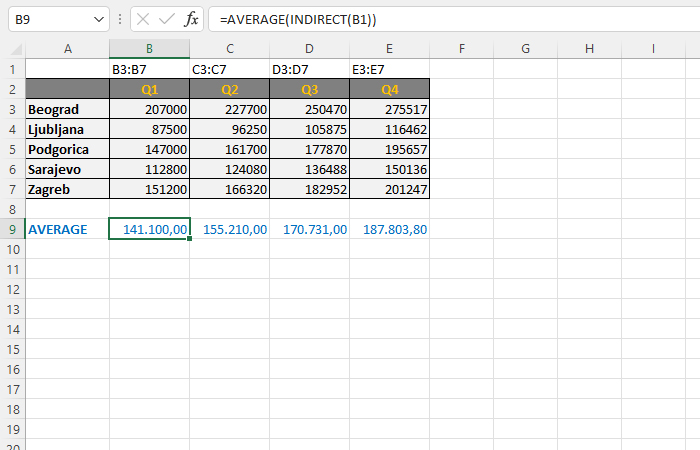# Formulas parameters

One of the earlier “recipes” was about the INDIRECT function. It serves to forward a range as a parameter of SUM, AVERAGE, MAX, MIN or, for example, some other aggregation function. A range is defined as string that is contained in another cell. Let’s show, in one simple example, how this works.

A table was created showing sales in four quarters by cities. How, using the parameter that defines the range of cells, to calculate the average sales?

Above each column that contains sales data in a quarter, we will enter the text that indicates the range of data on the basis of which we calculate the average. For instance in B1 we entered “B3:B7”, in C1 we added “C3:C7”, etc. Below the table, we have added a row in which the average values ​​are calculated. This is achieved using the formula:

=AVERAGE(INDIRECT(B1))

for sales values ​​in column B. By copying the formula we calculate the averages for the remaining columns as well. The INDIRECT function takes the string from the given cell and converts it into a range, and the range is passed on to the function.